##Conics through four points as triangle conics

Consider all conics passing through the four points {A,B,C,D}. Form the triangle ABC and the tripolar line L=tr(D) of D with respect to this triangle. Denote by (t) the generalized isogonal/isotomic transformation defined by these four points. Draw also the circum-conic (c0) and the in-conic (c1), both having perspector D.
Consider then an arbitrary conic cP passing through the five points {A,B,C,D,P}, P being a point on c0 considered as a parameter of the family of conics {cP}.

 All trilinear polars tr(X) of points X lying on such a conic are concurrent to a point P0 (the perspector of cP) lying on tr(D). In particular the trilinear polar of P passes through D.
 The trilinear polar L0=tr(P0) of P0 is tangent to the inconic c1 at a point P1. Last point as well as t(P0) are points collinear with line L1=PD.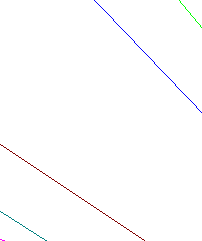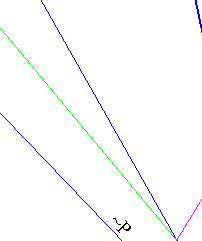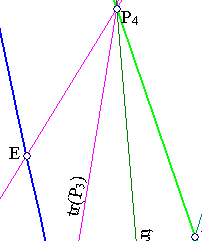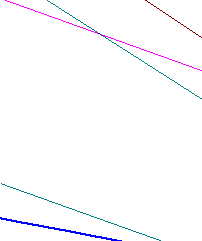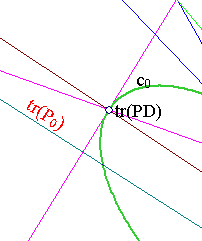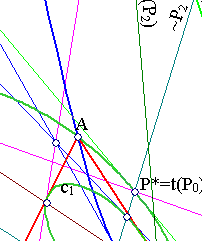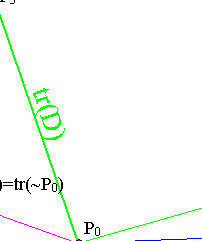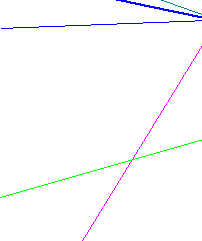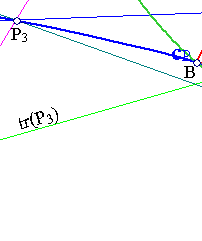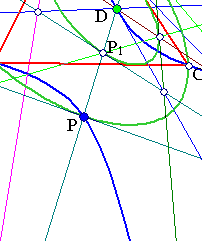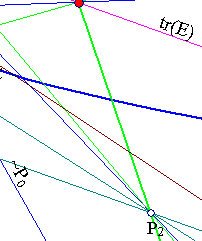Introduce the projective base with base points {A,B,C} and coordinator D. In this system the base points have correspondingly coordinates {(1,0,0),(0,1,0),(0,0,1)} and D has coordinates (1,1,1).
In this system also the trilinear polar tr(D) has an equation of the form x+y+z=0 and the conics (c0) and (c1) correspondingly have the equations (see IsogonalGeneralized.html ):
c0 : (1/x)+(1/y)+(1/z)=0,
c1 : x2+y2+z2-2yx-2zy-2xy=0.
An arbitrary conic cP through {A,B,C} has the equation:
a/x + b/y + c/z = 0 (1) .
D(1,1,1) assumed to be on the conic implies that
a+b+c=0 (2),
which means that P0(a,b,c) is a point on tr(D).
On the other side the trilinear polar of a point X(u,v,w) on the conic cP is
x/u + y/v + z/w = 0.
But a/u + b/v + c/w = 0 implies that this line passes through P0. This proves the claim in . The other claim follows from the fact that D is the perspector of the conic c0 hence all the tripolars of points on c0 pass through D.
To prove  notice that all points on tr(D) have the property that their trilinear polars are tangent to c1 (see IsotomicConicOfLine.html ). P0 having coordinates (a,b,c) implies that P*=t(P0) has coordinates (1/a,1/b,1/c), hence on the conic c0.
The intersection point of line P*+tD with c1 can be easily calculated to be (@ meaning cyclic permutet coordinates):
@(bc+2a2) (3).
This can be seen easily to satisfy the equation (1) of conic cp, hence coincides with P.
Note that t(P0) has coordinates (1/a,1/b,1/c) and is on c1. But this point satisfies also the equation of the conic ((1) and (2)), hence coincides with P.

Remark The figure suggests some other coincidences. Some of them are proved in the file IsotomicGeneral.html and again discussed in the file IsotomicChart.html .